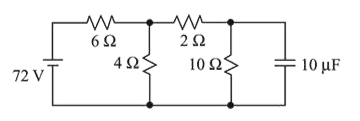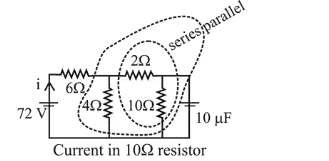# Determine the charge on the capacitor in the following circuit:

Question:

Determine the charge on the capacitor in the following circuit:1. (1) $60 \mu \mathrm{C}$

2. (2) $2 \mu \mathrm{C}$

3. (3) $10 \mu \mathrm{C}$

4. (4) $200 \mu \mathrm{C}$

Correct Option: , 4

Solution:

(4) At steady state, there is no current in capacitor.

$2 \Omega$ and $10 \Omega$ are in series. There equivalent resistance is $12 \Omega$. This $12 \Omega$ is in parallel with $4 \Omega$ and there combined resistance is $12 \times 4 /(12+4)$. This resistance is in series with $6 \Omega$. Therefore, current drawn from battery

$i=\frac{V}{R}=\left(\frac{72}{6+\frac{12 \times 4}{12+4}}\right)=8 A$$i^{\prime}=\left(\frac{4}{4+12}\right) 8=2 A$

Pd across capacitor, $\mathrm{V}=i^{\prime} \mathrm{R}=2 \times 10=20 \mathrm{~V}$

$\therefore \quad$ Charge on the capacitor, $\mathrm{q}=\mathrm{CV}$

$=10 \times 20=200 \mu \mathrm{C} .$